# Selina Solutions Concise Maths Class 10 Chapter 20 Cylinder, Cone and Sphere (Surface Area and Volume) Exercise 20(D)

A solid can be melted and recast into another solid. The initial volume and the final volume of the solids are the same. This exercise deals with such problems based on conversion of these solids. Students wishing to learn the correct steps for solving problems, then the Selina Solutions for Class 10 Maths is created for this purpose. Solutions of this exercise, Concise Selina Solutions for Class 10 Maths Chapter 20 Cylinder, Cone and Sphere (Surface Area and Volume) Exercise 20(D) are available in PDF format given below.

## Selina Solutions Concise Maths Class 10 Chapter 20 Cylinder, Cone and Sphere (Surface Area and Volume) Exercise 20(D) Download PDF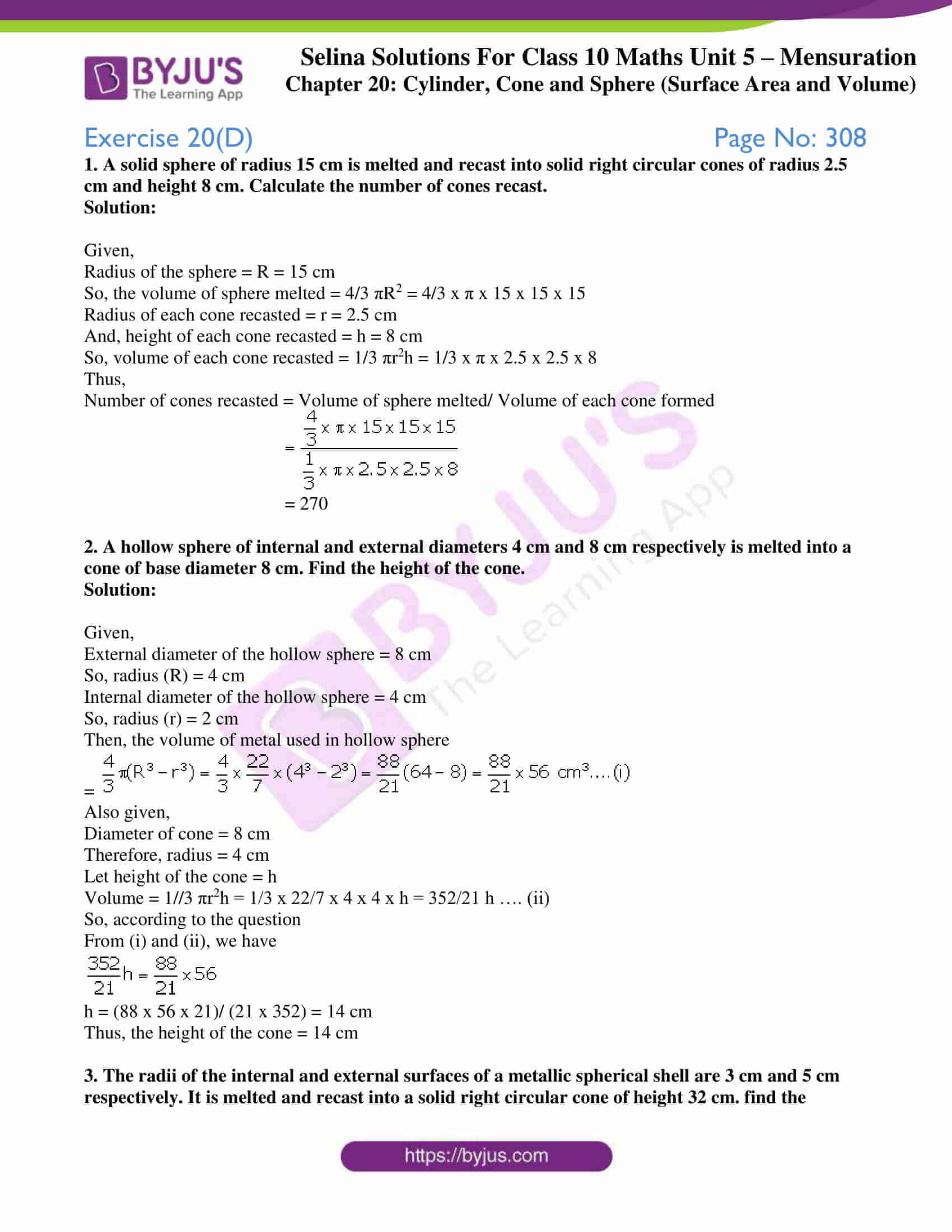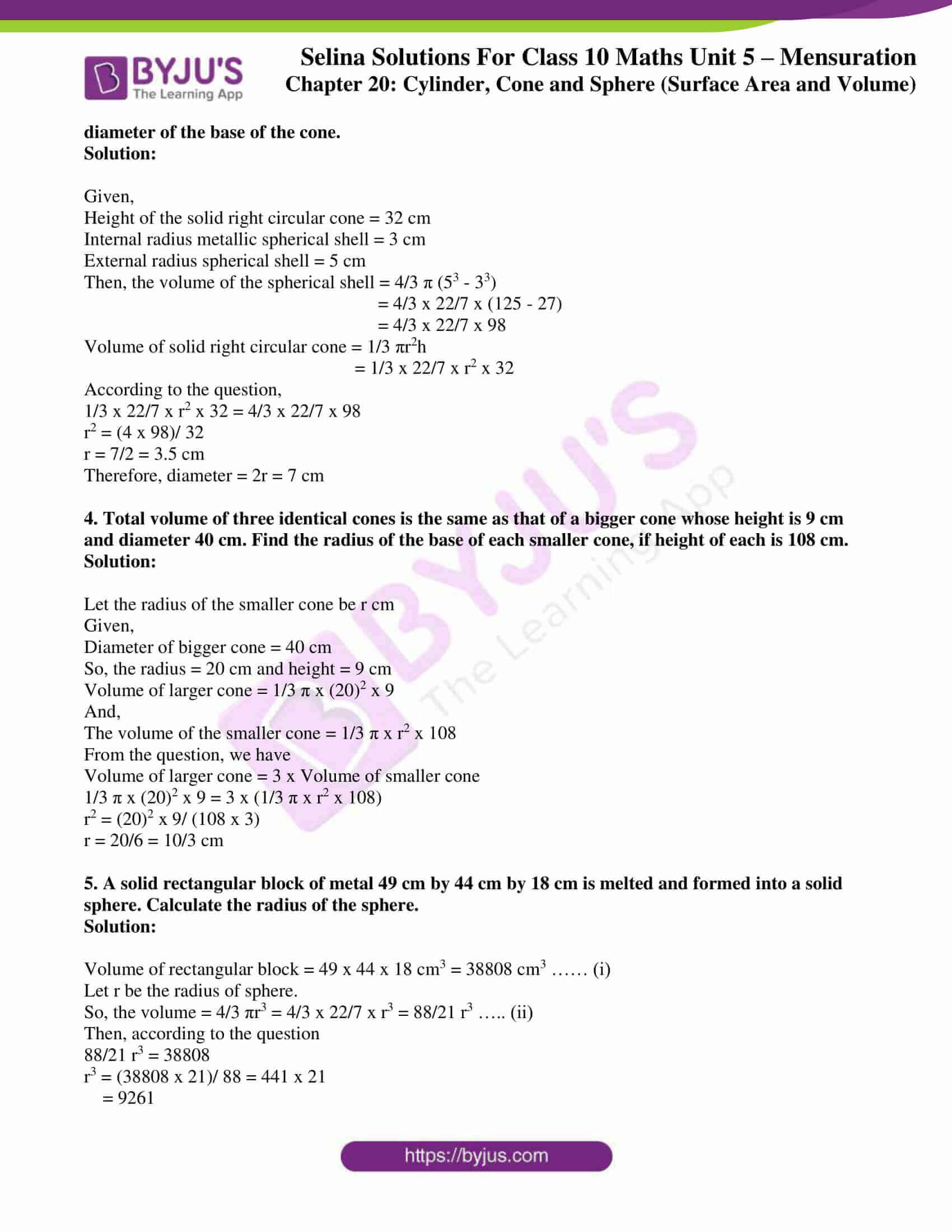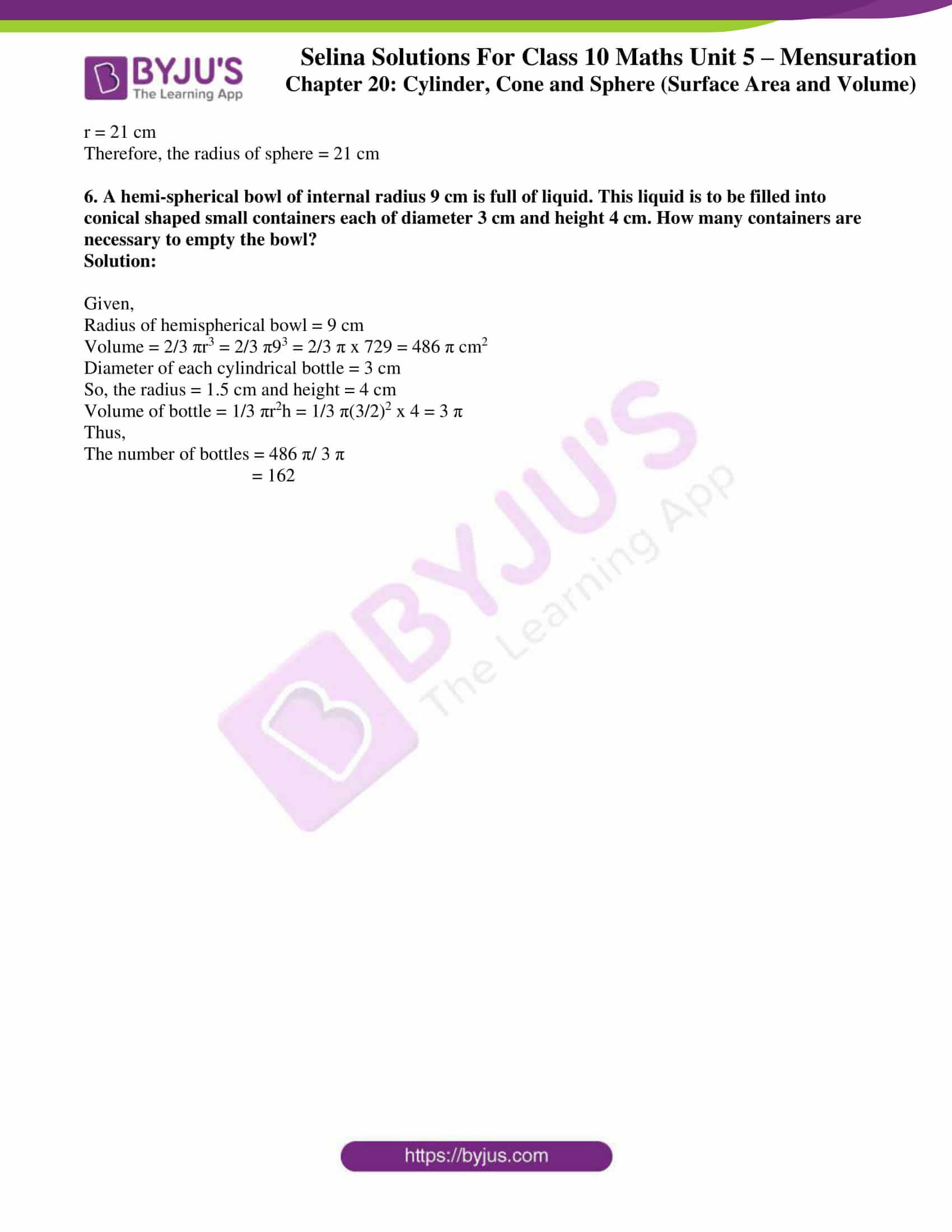### Access Selina Solutions Concise Maths Class 10 Chapter 20 Cylinder, Cone and Sphere (Surface Area and Volume) Exercise 20(D)

1. A solid sphere of radius 15 cm is melted and recast into solid right circular cones of radius 2.5 cm and height 8 cm. Calculate the number of cones recast.

Solution:

Given,

Radius of the sphere = R = 15 cm

So, the volume of sphere melted = 4/3 πR2 = 4/3 x π x 15 x 15 x 15

Radius of each cone recasted = r = 2.5 cm

And, height of each cone recasted = h = 8 cm

So, volume of each cone recasted = 1/3 πr2h = 1/3 x π x 2.5 x 2.5 x 8

Thus,

Number of cones recasted = Volume of sphere melted/ Volume of each cone formed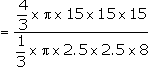= 270

2. A hollow sphere of internal and external diameters 4 cm and 8 cm respectively is melted into a cone of base diameter 8 cm. Find the height of the cone.

Solution:

Given,

External diameter of the hollow sphere = 8 cm

So, radius (R) = 4 cm

Internal diameter of the hollow sphere = 4 cm

So, radius (r) = 2 cm

Then, the volume of metal used in hollow sphere =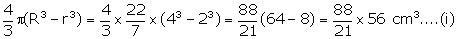Also given,

Diameter of cone = 8 cm

Let height of the cone = h

Volume = 1//3 πr2h = 1/3 x 22/7 x 4 x 4 x h = 352/21 h …. (ii)

So, according to the question

From (i) and (ii), we haveh = (88 x 56 x 21)/ (21 x 352) = 14 cm

Thus, the height of the cone = 14 cm

3. The radii of the internal and external surfaces of a metallic spherical shell are 3 cm and 5 cm respectively. It is melted and recast into a solid right circular cone of height 32 cm. find the diameter of the base of the cone.

Solution:

Given,

Height of the solid right circular cone = 32 cm

Internal radius metallic spherical shell = 3 cm

External radius spherical shell = 5 cm

Then, the volume of the spherical shell = 4/3 π (53 – 33)

= 4/3 x 22/7 x (125 – 27)

= 4/3 x 22/7 x 98

Volume of solid right circular cone = 1/3 πr2h

= 1/3 x 22/7 x r2 x 32

According to the question,

1/3 x 22/7 x r2 x 32 = 4/3 x 22/7 x 98

r2 = (4 x 98)/ 32

r = 7/2 = 3.5 cm

Therefore, diameter = 2r = 7 cm

4. Total volume of three identical cones is the same as that of a bigger cone whose height is 9 cm and diameter 40 cm. Find the radius of the base of each smaller cone, if height of each is 108 cm.

Solution:

Let the radius of the smaller cone be r cm

Given,

Diameter of bigger cone = 40 cm

So, the radius = 20 cm and height = 9 cm

Volume of larger cone = 1/3 π x (20)2 x 9

And,

The volume of the smaller cone = 1/3 π x r2 x 108

From the question, we have

Volume of larger cone = 3 x Volume of smaller cone

1/3 π x (20)2 x 9 = 3 x (1/3 π x r2 x 108)

r2 = (20)2 x 9/ (108 x 3)

r = 20/6 = 10/3 cm

5. A solid rectangular block of metal 49 cm by 44 cm by 18 cm is melted and formed into a solid sphere. Calculate the radius of the sphere.

Solution:

Volume of rectangular block = 49 x 44 x 18 cm3 = 38808 cm3 …… (i)

Let r be the radius of sphere.

So, the volume = 4/3 πr3 = 4/3 x 22/7 x r3 = 88/21 r3 ….. (ii)

Then, according to the question

88/21 r3 = 38808

r3 = (38808 x 21)/ 88 = 441 x 21

= 9261

r = 21 cm

Therefore, the radius of sphere = 21 cm

6. A hemi-spherical bowl of internal radius 9 cm is full of liquid. This liquid is to be filled into conical shaped small containers each of diameter 3 cm and height 4 cm. How many containers are necessary to empty the bowl?

Solution:

Given,

Radius of hemispherical bowl = 9 cm

Volume = 2/3 πr3 = 2/3 π93 = 2/3 π x 729 = 486 π cm2

Diameter of each cylindrical bottle = 3 cm

So, the radius = 1.5 cm and height = 4 cm

Volume of bottle = 1/3 πr2h = 1/3 π(3/2)2 x 4 = 3 π

Thus,

The number of bottles = 486 π/ 3 π

= 162